Courses

# RD Sharma Solutions (Part - 1) - Ex-21.2, Mensuration - II Area of Circle, Class 7, Math Class 7 Notes | EduRev

## Class 7: RD Sharma Solutions (Part - 1) - Ex-21.2, Mensuration - II Area of Circle, Class 7, Math Class 7 Notes | EduRev

The document RD Sharma Solutions (Part - 1) - Ex-21.2, Mensuration - II Area of Circle, Class 7, Math Class 7 Notes | EduRev is a part of the Class 7 Course RD Sharma Solutions for Class 7 Mathematics.
All you need of Class 7 at this link: Class 7

#### Question 1:

Find the area of a circle whose radius is
(i) 7 cm
(ii) 2.1 m
(iii) 7 km

(i) We know that the area A of a circle of radius is given by A = πr2πr2.
Here, r  = 7 cm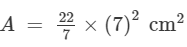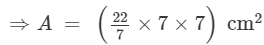= (22×7) cm2 = 154 cm2.

(ii) We know that the area A of a circle of radius r is given by A = πr2
Here, r  = 2.1 m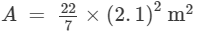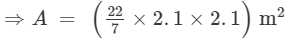= (22×0.3×2.1) m2 = 13.86 m2

(iii) We know that the area A of a circle of radius r is given by A =  πr2πr2.
Here, r  = 7 km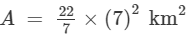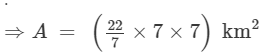= (22×7) km2 = 154 km2

#### Question 2:

Find the area of a circle whose diameter is
(i) 8.4 cm
(ii) 5.6 m
(iii) 7 km

(i) Let r be the radius of the circle. Then, r = 8.4 ÷ 2 = 4.2 cm.
∴ Area of the circle  = πr2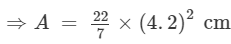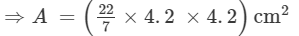= (22×0.6×4.2) cm2 = 55.44 cm2.

(ii) Let r be the radius of the circle. Then, r = 5.6 ÷ 2 = 2.8 m.
Area of the circle  = πr2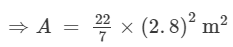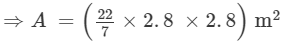= (22×0.4×2.8) m2 = 24.64 m2.

(iii) Let r be the radius of the circle. Then, r = 7 ÷ 2 = 3.5 km.
Area of the circle  = πr2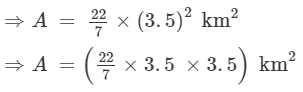= (22×0.5×3.5) km2 = 38.5 km2

#### Question 3:

The area of a circle is 154 cm2. Find the radius of the circle.

Let the radius of the circle be r cm.
Area of the circle (A) = 154 cm2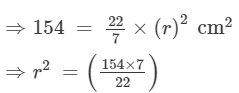⇒r2 =(1078/22)
⇒r2 =(49)
⇒r =7 cm.

Hence, the radius of the circle is 7 cm.

#### Question 4:

Find the radius of a circle, if its area is
(i) 4 π cm2
(ii) 55.44 m2
(iii) 1.54 km2

(i) Let the radius of the circle be r cm.

∴ Area of the circle (A) = 4π cm2

⇒4π = π×(r)2 cm2
⇒r2 =(4π/π)  = 4
⇒r =2 cm.

(ii) Let the radius of the circle be r cm.

∴ Area of the circle (A) = 55.44 m2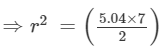⇒r2 =(17.64)
⇒r =4.2 m

(iii) Let the radius of the circle be r cm.

∴ Area of the circle (A) = 1.54 km2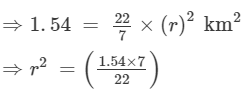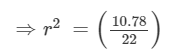⇒r2 =(0.49)
⇒r =0.7 km = 700 m

#### Question 5:

The circumference of a circle is 3.14 m, find its area.

We have :

Circumference of the circle = 3.14  m = 2πr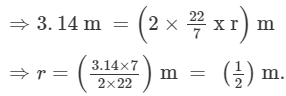Area of the circle (A) = πr2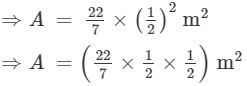= (22/28) m2 = 0.785 m2.

#### Question 6:

If the area of a circle is 50.24 m2, find its circumference.

We have :
Area of the circle (A) = πr2 = 50.24 m2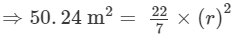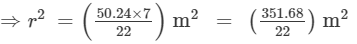=  15.985 m2

⇒r = 3.998 m.

Circumference of circle (C) = 2πr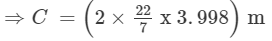⇒ C=(44×0.571) m = 25.12 m.

#### Question 7:

A horse is tied to a pole with 28 m long string. Find the area where the horse can graze. (Take π = 22/7).

We have :
Length of the string = 28 m
The area over which the horse can graze is the same as the area of a circle of radius 28 m.
Hence, required area = πr2 =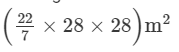= (22×4×28×)m2 = (22×4×28×)m2 = 2464 m2.

#### Question 8:

A steel wire when bent in the form of a square encloses an area of 121 cm2. If the same wire is bent in the form of a circle, find the area of the circle.

We have :
Area of the square = 121 cm2
⇒ (side)2 = (11)2 cm2
⇒ side =  11 cm.
So, the perimeter of the square = 4(side) = (4 x 11) cm = 44 cm.

Let be the radius of the circle. Then,
Circumference of the circle = Perimeter of the square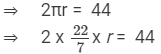⇒ r = 7 cm.
∴ Area of the circle = πr2 =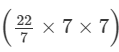= 22×7 = 154 cm2

#### Question 9:

A road which is 7 m wide surrounds a circular park whose circumference is 352 m. Find the area of of road.

We have :
Circumference of the circular park = 2πr = 352 m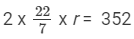⇒ r = 56 m.
Radius of the path including the 7m wide road = (r +7) =  56 +7 = 63 m.
∴ Area of the road :
=[π(63)2 − π(56)2] m2
=π[(63)2 − (56)2] m2
=π[(63 +56)(63 − 56)] m2
=π[(119)(7)]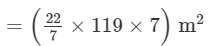= (22×119) m2 = 2618 m2

#### Question 10:

Prove that the area of a circular path of uniform width h surrounding a circular region of radius r is πh (2r + h).

Radius  of the circular region = r
Radius of the circular path of uniform width h surrounding the circular region of radius r = (r + h).
∴ Area of the path
=[π(r+h)2πr2]
=π[(r+h)2r2]
=π[r2 + 2rh +h2 r2]
=π[2rh+h2]=πh[2r+h]

The document RD Sharma Solutions (Part - 1) - Ex-21.2, Mensuration - II Area of Circle, Class 7, Math Class 7 Notes | EduRev is a part of the Class 7 Course RD Sharma Solutions for Class 7 Mathematics.
All you need of Class 7 at this link: Class 7Use Code STAYHOME200 and get INR 200 additional OFF Use Coupon Code
All Tests, Videos & Notes of Class 7: Class 7

### Up next### Top Courses for Class 7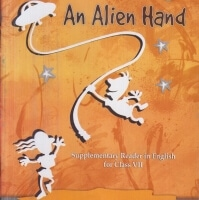## RD Sharma Solutions for Class 7 Mathematics

97 docs

### Up next### Top Courses for Class 7Track your progress, build streaks, highlight & save important lessons and more!

,

,

,

,

,

,

,

,

,

,

,

,

,

,

,

,

,

,

,

,

,

,

,

,

,

,

,

,

,

,

;